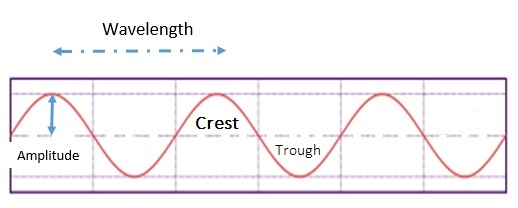# Introduction to Wave Description

The main characteristics of a wave are as follows:• Crests are the high points.
• Troughs are the low points.
• Equilibrium position is the dashed line in the figure which represents the midpoint of the vibration.
• Wavelength (λ) is the distance between any successive identical parts of the wave.
For example the distance from one crest to the next crest.
• Amplitude is the distance from the midpoint to the crest (or trough) of the wave.
Basically, amplitude represents maximum displacement from equilibrium.
• Frequency (f) is the number of up-and-down vibrations an object makes in a given time (usually in one second).
A complete one up-and-down cycle is one vibration.
• Frequency is measured in hertz (Hz).
• A frequency of 1 Hz is a vibration that occurs once every second.
• Sound has a frequency of a few 100 or 1000 Hz.
• Radio waves have frequencies of a few million Hz (MHz). Like radio stations.
• Cell phones operate at few billon Hz (GHz).
• Period is the time for one complete vibration.
• Frequency (vibrations per second) and Period (seconds per vibration) are reciprocals.
Frequency = 1 / Period ⇒ Period = 1 / Frequency
f = 1 / TT = 1 / f

### Exercise-1

Exercise-1 on Waves

### Exercise-2

Exercise-2 on Waves

### Exercise-3

Exercise-3 on Waves